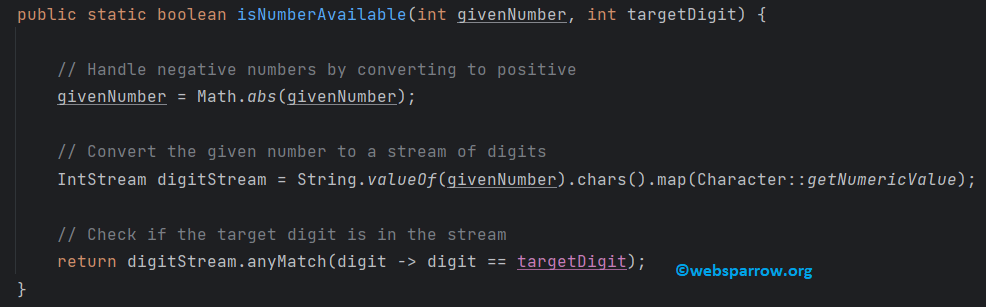# Finding a Specific Digit in a Number using Java

On this page, we’ll explore a simple yet common problem: finding a specific digit in a given number using Java. We’ll cover both traditional and Java 8 stream-based approaches to solve this problem.Let’s start with a traditional approach using loops and basic arithmetic. We’ll write a Java method to find a specific digit within a given number.

FindDigit.java
``````package org.websparrow;

public class FindDigit {

public static void main(String[] args) {
int givenNumber = 12345678;
int targetDigit = 5;
int targetDigit2 = 32;

if (isNumberAvailable(givenNumber, targetDigit)) {
System.out.println(targetDigit + " is available in " + givenNumber);
} else {
System.out.println(targetDigit + " is not available in " + givenNumber);
}

if (isNumberAvailable(givenNumber, targetDigit2)) {
System.out.println(targetDigit2 + " is available in " + givenNumber);
} else {
System.out.println(targetDigit2 + " is not available in " + givenNumber);
}
}

public static boolean isNumberAvailable(int givenNumber, int targetDigit) {
// Handle negative numbers by converting to positive
givenNumber = Math.abs(givenNumber);

// Check if the target digit is zero (special case)
if (targetDigit == 0 && givenNumber == 0) {
return true;
}

// Iterate through each digit of the given number
while (givenNumber > 0) {
int digit = givenNumber % 10; // Extract the last digit
if (digit == targetDigit) {
return true; // Found a match
}
givenNumber /= 10; // Remove the last digit
}

// If the loop finishes without finding a match, return false
return false;
}
}``````

In this approach, we first handle negative numbers by converting them to positive. Then, we use a `while` loop to extract each digit from the given number and check if it matches the target digit. This loop continues until we’ve checked all the digits. If we find a match, we return `true`; otherwise, we return `false`.

Output:

console.log
``````5 is available in 12345678
32 is not available in 12345678``````

## 2. Java 8 Stream Approach

Now, let’s explore a more modern and concise way to solve this problem using Java 8 streams:

FindDigitJava8.java
``````package org.websparrow.java8;

import java.util.stream.IntStream;

public class FindDigitJava8 {

public static void main(String[] args) {
int givenNumber = 12345678;
int targetDigit = 5;
int targetDigit2 = 32;

if (isNumberAvailable(givenNumber, targetDigit)) {
System.out.println(targetDigit + " is available in " + givenNumber);
} else {
System.out.println(targetDigit + " is not available in " + givenNumber);
}

if (isNumberAvailable(givenNumber, targetDigit2)) {
System.out.println(targetDigit2 + " is available in " + givenNumber);
} else {
System.out.println(targetDigit2 + " is not available in " + givenNumber);
}
}

public static boolean isNumberAvailable(int givenNumber, int targetDigit) {
// Handle negative numbers by converting to positive
givenNumber = Math.abs(givenNumber);

// Convert the given number to a stream of digits
IntStream digitStream = String.valueOf(givenNumber).chars().map(Character::getNumericValue);

// Check if the target digit is in the stream
return digitStream.anyMatch(digit -> digit == targetDigit);
}
}``````

In this Java 8 approach, we use streams to perform the digit search. First, we convert the given number to a string, and then to a stream of characters. We map each character to its numeric value using `Character::getNumericValue`. Finally, we use `anyMatch` to check if the target digit is present in the stream.

Output:

console.log
``````5 is available in 12345678
32 is not available in 12345678``````

## Conclusion

In this blog post, we’ve explored two different approaches to finding a specific digit in a number using Java. The traditional approach provides a detailed, step-by-step solution that works efficiently. On the other hand, the Java 8 stream-based approach offers a more concise and modern solution.

## References

#### Similar Posts